Download Presentation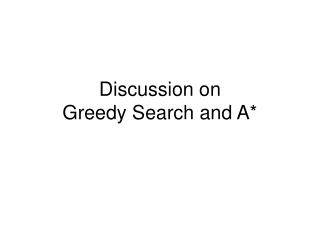Discussion on Greedy Search and A*

# Discussion on Greedy Search and A* - PowerPoint PPT Presentation

Discussion on Greedy Search and A*. Best-first search. Idea: use an evaluation function f(n) for each node estimate of "desirability" Expand most desirable unexpanded node Implementation : Order the nodes in fringe in decreasing order of desirability Special cases:I am the owner, or an agent authorized to act on behalf of the owner, of the copyrighted work described.
Download Presentation## Discussion on Greedy Search and A*

An Image/Link below is provided (as is) to download presentation

Download Policy: Content on the Website is provided to you AS IS for your information and personal use and may not be sold / licensed / shared on other websites without getting consent from its author.While downloading, if for some reason you are not able to download a presentation, the publisher may have deleted the file from their server.

- - - - - - - - - - - - - - - - - - - - - - - - - - E N D - - - - - - - - - - - - - - - - - - - - - - - - - -
Presentation Transcript### Discussion on Greedy Search and A*Best-first search
• Idea: use an evaluation functionf(n) for each node
• estimate of "desirability"
• Expand most desirable unexpanded node
• Implementation:

Order the nodes in fringe in decreasing order of desirability

• Special cases:
• greedy best-first search
• A* search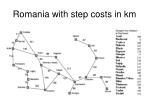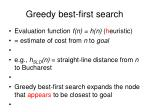Greedy best-first search
• Evaluation function f(n) = h(n) (heuristic)
• = estimate of cost from n to goal
• e.g., hSLD(n) = straight-line distance from n to Bucharest
• Greedy best-first search expands the node that appears to be closest to goal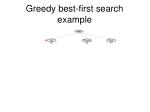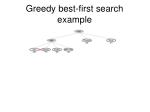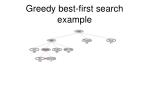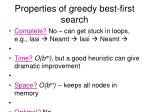Properties of greedy best-first search
• Complete? No – can get stuck in loops, e.g., Iasi  Neamt  Iasi  Neamt 
• Time?O(bm), but a good heuristic can give dramatic improvement
• Space?O(bm) -- keeps all nodes in memory
• Optimal? No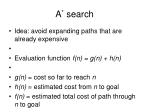A* search
• Idea: avoid expanding paths that are already expensive
• Evaluation function f(n) = g(n) + h(n)
• g(n) = cost so far to reach n
• h(n) = estimated cost from n to goal
• f(n) = estimated total cost of path through n to goal• A heuristic h(n) is admissible if for every node n,

h(n) ≤ h*(n), where h*(n) is the true cost to reach the goal state from n.

• An admissible heuristic never overestimates the cost to reach the goal, i.e., it is optimistic
• Example: hSLD(n) (never overestimates the actual road distance)
• Theorem: If h(n) is admissible, A* using TREE-SEARCH is optimalOptimality of A* (proof)

• Suppose some suboptimal goal G2has been generated and is in the fringe. Let n be an unexpanded node in the fringe such that n is on a shortest path to an optimal goal G.
• f(G2) = g(G2) since h(G2) = 0
• g(G2) > g(G) since G2 is suboptimal
• f(G) = g(G) since h(G) = 0
• f(G2) > f(G) from aboveOptimality of A* (proof)
• Suppose some suboptimal goal G2has been generated and is in the fringe. Let n be an unexpanded node in the fringe such that n is on a shortest path to an optimal goal G.
• f(G2) > f(G) from above
• h(n) ≤ h*(n) since h is admissible
• g(n) + h(n) ≤ g(n) + h*(n)
• f(n) ≤ f(G)

Hence f(G2) > f(n), and A* will never select G2 for expansion; Contradiction: Algorithm would have not terminated at G2 and expanded n instead because f(n)<f(G)f(G2)Consistent heuristics
• A heuristic is consistent if for every node n, every successor n' of n generated by any action a,

h(n) ≤ c(n,a,n') + h(n')

• If h is consistent, we have

f(n') = g(n') + h(n')

= g(n) + c(n,a,n') + h(n')

≥ g(n) + h(n)

= f(n)

• i.e., f(n) is non-decreasing along any path.
• Theorem: If h(n) is consistent, A* using GRAPH-SEARCH is optimalOptimality of A*
• A* expands nodes in order of increasing f value
• Contour i has all nodes with f=fi, where fi < fi+1Properties of A\$^*\$
• Complete? Yes (unless there are infinitely many nodes with f ≤ f(G) )
• Time? Exponential
• Space? Keeps all nodes in memory
• Optimal? YesE.g., for the 8-puzzle:

• h1(n) = number of misplaced tiles
• h2(n) = total Manhattan distance

(i.e., no. of squares from desired location of each tile)

• h1(S) = ? 8
• h2(S) = ? 3+1+2+2+2+3+3+2 = 18Dominance
• If h2(n) ≥ h1(n) for all n (both admissible)
• then h2dominatesh1
• h2is better for search
• Typical search costs (average number of nodes expanded):
• d=12 IDS = 3,644,035 nodes A*(h1) = 227 nodes A*(h2) = 73 nodes
• d=24 IDS = too many nodes A*(h1) = 39,135 nodes A*(h2) = 1,641 nodes